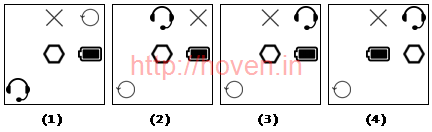# Image Series Quiz Set 007

### Question 1

Which image would be the next image if the pattern is continued?

Question Image:A

1.

B

2.

C

3.

D

4.

Soln.
Ans: a

The pattern starts with four icons. On each step it rotates by 90° anti-clockwise and replaces one of the original icons with a new one.

### Question 2

Which image would be the next image if the pattern is continued?

Question Image:A

1.

B

2.

C

3.

D

4.

Soln.
Ans: a

In each figure, the number of small circles varies between one and two alternately. The small circles also move in an anticlockwise direction. The shaded area moves in the clockwise direction.

### Question 3

Which image would be the next image if the pattern is continued?

Question Image:A

1.

B

2.

C

3.

D

4.

Soln.
Ans: d
```1   2   3
=========
4   5   6
=========
7   8   9
```

With reference to the above diagram, on each step the movements are as shown below.

1. 1 moves to 2
2. 2 moves to 3
3. 3 moves to 4
4. 4 moves to 5
5. 5 moves to 6
6. 6 moves to 7
7. 7 moves to 8
8. 8 moves to 9
9. 9 moves to 1

The icons are cyclically shifting.

### Question 4

Which image would be the next image if the pattern is continued?

Question Image:A

1.

B

2.

C

3.

D

4.

Soln.
Ans: c

Triangle rotates 45° anticlockwise, whereas the T moves 90° anticlockwise.

### Question 5

Which image would be the next image if the pattern is continued?

Question Image:A

1.

B

2.

C

3.

D

4.

Soln.
Ans: c
```1   2   3
=========
4   5   6
=========
7   8   9
```

With reference to the above diagram, on each step the movements are as shown below.

1. 6 moves to 5
2. 5 moves to 2
3. 2 moves to 3
4. 3 moves to 7
5. 7 moves to 6